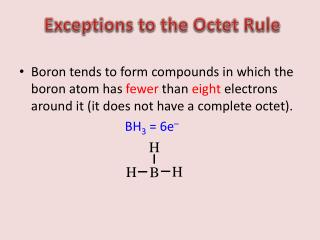Download PresentationExceptions to the Octet Rule

# Exceptions to the Octet Rule - PowerPoint PPT PresentationDownload Presentation## Exceptions to the Octet Rule

- - - - - - - - - - - - - - - - - - - - - - - - - - - E N D - - - - - - - - - - - - - - - - - - - - - - - - - - -
##### Presentation Transcript

1. Exceptions to the Octet Rule • Boron tends to form compounds in which the boron atom has fewer than eight electrons around it (it does not have a complete octet). • BH3 = 6e–

2. When it is necessary to exceed the octet rule for one of several third-row (or higher) elements, place the extra electrons on the central atom. • SF4 = 34e– AsBr5 = 40e–

3. Exceptions to the Octet Rule • C, N, O, and F should always be assumed to obey the octet rule. • B and Be often have fewer than 8 electrons around them in their compounds. • Second-row elements never exceed the octet rule. • Third-row and heavier elements often satisfy the octet rule but can exceed the octet rule by using their empty valence d orbitals.

4. When writing the Lewis structure for a molecule, satisfy the octet rule for the atoms first. If electrons remain after the octet rule has been satisfied, then place them on the elements having availabled orbitals (elements in Period 3 or beyond).

5. Resonance • Sometimes more than one valid Lewis structure (one that obeys the rules outlined) is possible for a given molecule. • Actual structure is an average of the resonance structures. • Electrons are really delocalized – they can move around the entire molecule. ATOMS do not move!

6. Formal Charge • At times there are several possible Lewis structures for the same molecule. • Formal charges are used to evaluate nonequivalent Lewis structures. (move the ATOMS, not electrons) • Atoms in molecules try to achieve formal charges as close to zero as possible. • Any negative formal charges are expected to reside on the most electronegative atoms.

7. H H C O H C O H Two possible skeletal structures of formaldehyde (CH2O) • Which one is the correct Lewis structure? • To determine the correct structure, the charge on each atom in the various Lewis structures must be estimated and those charges are used to select the most appropriate structure. • The formal charge of an atom in a molecule is the difference between the number of valence electrons on the free atom and the number of valence electrons assigned to the atom in the molecule.

8. To determine the formal charge of a given atom in a molecule, we need to know two things. • The number of valence electrons on the free neutral atom (which has zero net charge because the number of electrons equals the number of protons). • The number of valence electrons “belonging” to the atom in a molecule. • If in the molecule the atom has the same number of valence electrons as it does in the free state, the (+) and (-) charges balance and it has a formal charge of zero. • If the atom has one more valence electron in a molecule than it has as a free atom, it has a formal charge of -1, and so on.

9. formal charge on an atom in a Lewis structure total number of valence electrons in the free atom total number of nonbonding electrons ( total number of bonding electrons ) 1 - - = 2 The sum of the formal charges of the atoms in a molecule or ion must equal the charge on the molecule or ion. • Make the following assumptions: • Lone pair electrons belong entirely to the atom in question. • Shared electrons are divided equally between the two sharing atoms.

10. H C O H C – 4 e- O – 6 e- 2H – 2x1 e- 12 e- formal charge on an atom in a Lewis structure total number of valence electrons in the free atom total number of nonbonding electrons ( total number of bonding electrons ) 1 - - = 2 single bonds (2x2) = 4 2 1 double bond = 4 2 lone pairs (2x2) = 4 Total = 12 -1 +1 formal charge on C = 4 - 2- ½ x 6 = -1 formal charge on O = 6 - 2- ½ x 6 = +1

11. C – 4 e- H O – 6 e- C O H 2H – 2x1 e- 12 e- formal charge on an atom in a Lewis structure total number of valence electrons in the free atom total number of nonbonding electrons ( total number of bonding electrons ) 1 - - = 2 single bonds (2x2) = 4 2 1 double bond = 4 2 lone pairs (2x2) = 4 Total = 12 0 0 formal charge on C = 4 - 0- ½ x 8 = 0 formal charge on O = 6 - 4- ½ x 4 = 0

12. Which is the most likely Lewis structure for CH2O? H C O H H C O H -1 +1 0 0 Formal Charge and Lewis Structures • For neutral molecules, a Lewis structure in which there are no formal charges is preferable to one in which formal charges are present. • Lewis structures with large formal charges are less plausible than those with small formal charges. • Among Lewis structures having similar distributions of formal charges, the most plausible structure is the one in which negative formal charges are placed on the more electronegative atoms.